# Class 9 NCERT Solutions- Chapter 12 Heron’s Formula – Exercise 12.1

### Question 1. A traffic signal board, indicating ‘SCHOOL AHEAD’, is an equilateral triangle with side ‘a’. Find the area of the signal board, using Heron’s formula. If its perimeter is 180 cm, what will be the area of the signal board?

Solution:

As it is mentioned here that, ΔABC is an equilateral triangle having side length = a.

So, here

AB =BC = AC = a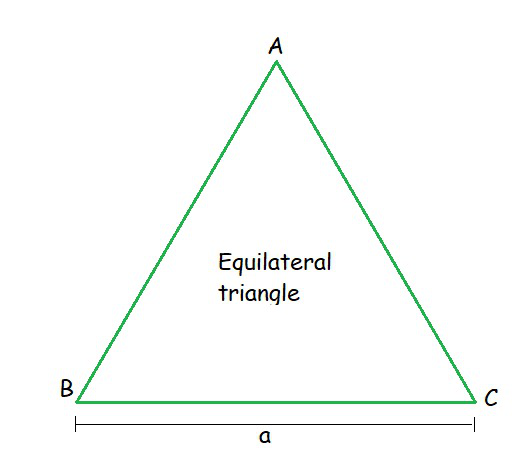Perimeter of Equilateral triangle = 3× (Length of a side)

= 3×a = 3a

and perimeter = 180 cm (given)

So, 3a = 180

a = 60 cm.

Hence, length of each side is 60 cm.

Now, Area of △ABC can be calculated by Heron’s Formula, where

AB = a = 60 cm

BC = b = 60 cm

AC = c = 60 cm

Semi Perimeter (s) = (a+b+c)/2

s = 180/2

s = 90 cm

ar(△ABC) = √s(s-a)(s-b)(s-c)

= √90(90-60)(90-60)(90-60)

= √90×(30)×(30)×(30)

= 900√3 cm2

Hence, the area of the signal board = 900√3 cm2

### Question 2. The triangular side walls of a flyover have been used for advertisements. The sides of the walls are 122 m, 22 m and 120 m (see Fig.). The advertisements yield an earning of ₹ 5000 per m2 per year. A company hired one of its walls for 3 months. How much rent did it pay?

Solution: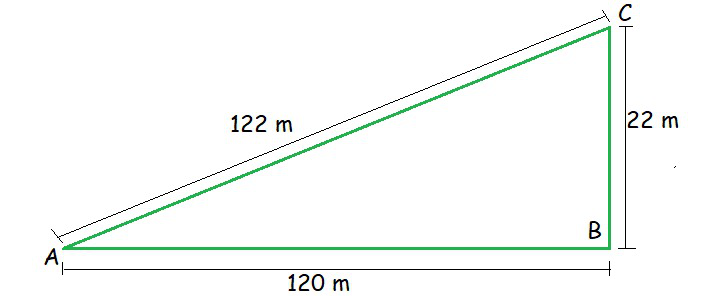Here, Area of △ABC can be calculated by Heron’s Formula, where

AB = a = 120 m

BC = b = 22 m

AC = c = 122 m

Semi Perimeter (s) = (a+b+c)/2

s = (120+22+122)/2

s = 132 m

ar(△ABC) = √s(s-a)(s-b)(s-c)

= √132(132-120)(132-22)(132-122)

= √132×(12)×(110)×(10)

= 1320 m2

As it is given that,

For 1 year we cost

1 m2 = ₹ 5000

So, for 3 months,

1 m2 = ₹ 5000 × (1/4)

For area of walls 1320 m2 = 5000×(1/4)×(1320)

= ₹ 16,50,000

Hence, ₹ 16,50,000 much rent company will be pay for 3 months.

### Question 3. There is a slide in a park. One of its side walls has been painted in some colour with a message “KEEPTHE PARK GREEN AND CLEAN” (see Fig.). If the sides of the wall are 15 m, 11 m, and 6 m, find the area painted in colour.

Solution: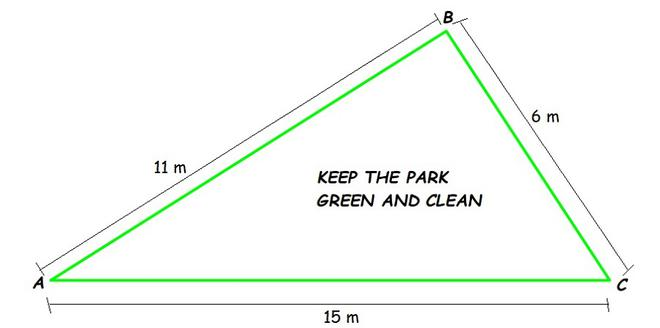Here, Area of △ABC can be calculated by Heron’s Formula, where

AB = a = 11 m

BC = b = 6 m

AC = c = 15 m

Semi Perimeter (s) = (a+b+c)/2

s = (11+6+15)/2

s = 16 m

ar(△ABC) = √s(s-a)(s-b)(s-c)

= √16(16-11)(16-6)(16-15)

= √16×(5)×(10)×(1)

= 20√2 m2

Hence, the area painted in colour is 20√2 m2

### Question 4. Find the area of a triangle two sides of which are 18 cm and 10 cm and the perimeter is 42 cm.

Solution:

Here, length of two sides are given as 18 cm and 10 cm respectively.

and, perimeter = 42 cm.

Hence, length of third side = (Perimeter)-(length of two side)

= 42-(18+10)

AC = 14 cm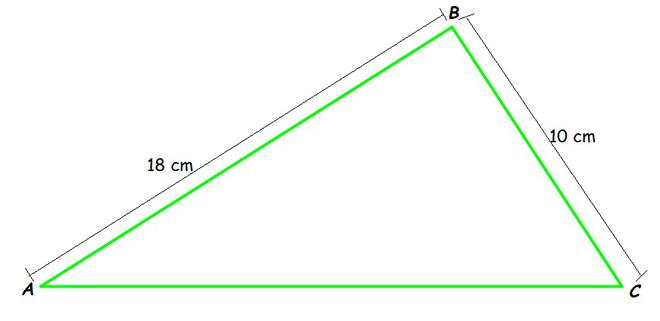Here, Area of △ABC can be calculated by Heron’s Formula, where

AB = a = 18 cm

BC = b = 10 cm

AC = c = 14 cm

Semi Perimeter (s) = (a+b+c)/2

s = (18+10+14)/2

s = 21 cm

ar(△ABC) = √s(s-a)(s-b)(s-c)

= √21(21-18)(21-10)(21-14)

= √21×(3)×(11)×(7)

= 21√11 cm2

Hence, the area of triangle is 21√11 cm2

### Question 5. Sides of a triangle are in the ratio of 12:17:25 and its perimeter is 540 cm. Find its area.

Solution:

The ratio of the sides of the triangle are given as 12 : 17 : 25

Lets consider the common ratio between the sides of the triangle be p

Then, the sides are 12p, 17p and 25p

The perimeter of the triangle = 540 cm  (Given)

12p+17p+25p = 540 cm

54p = 540cm

So, p = 10

Hence, the sides of triangle are 120 cm, 170 cm, 250 cm.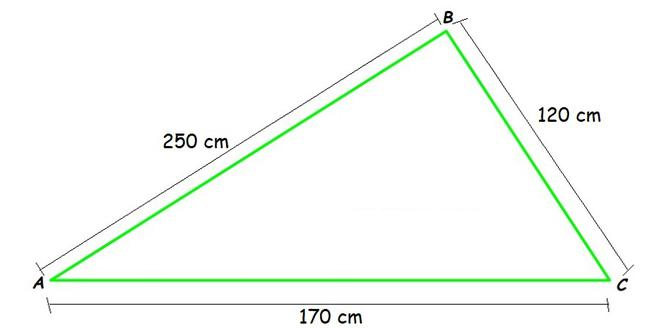Here, Area of △ABC can be calculated by Heron’s Formula, where

AB = a = 250 cm

BC = b = 120 cm

AC = c = 170 cm

Semi Perimeter (s) = (a+b+c)/2

s = (250+120+170)/2

s = 270 cm

ar(△ABC) = √s(s-a)(s-b)(s-c)

= √270(270-250)(270-120)(270-170)

= √270×(20)×(150)×(100)

= 9000 cm2

Hence, the area of triangle is 9000 cm2 .

### Question 6. An isosceles triangle has perimeter 30 cm and each of the equal sides is 12 cm. Find the area of the triangle.

Solution:

Here, length of two equal sides of isosceles triangle are given as 12 cm.

and, perimeter = 30 cm.

Hence, length of third side = (Perimeter)-(length of two side)

= 30-(12+12)

AC = 6 cm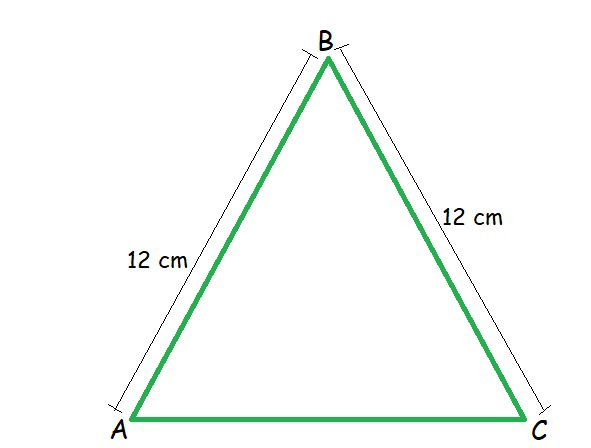Here, Area of △ABC can be calculated by Heron’s Formula, where

AB = a = 12 cm

BC = b = 12 cm

AC = c = 6 cm

Semi Perimeter (s) = (a+b+c)/2

s = (12+12+6)/2

s = 15 cm

ar(△ABC) = √s(s-a)(s-b)(s-c)

= √15(15-12)(15-12)(15-6)

= √15×(3)×(3)×(9)

= 9√15 cm2

Hence, the area of triangle is 9√15 cm2

Whether you're preparing for your first job interview or aiming to upskill in this ever-evolving tech landscape, GeeksforGeeks Courses are your key to success. We provide top-quality content at affordable prices, all geared towards accelerating your growth in a time-bound manner. Join the millions we've already empowered, and we're here to do the same for you. Don't miss out - check it out now!

Previous
Next